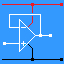RANDOM PAGE

SITE SEARCH

LOG
IN

HELP

This is the AQA version closing after June 2019. Visit the the version for Eduqas instead.

a

## Tuned Circuit PropertiesTuned circuits consist of an inductor coil and a capacitor (often variable). The examples in the image are designed for high transmitter voltages. Much smaller ones are used in receivers. A tuned circuit has a very high impedance at its resonant frequency (ideally = infinity). At other frequencies, its impedance is lower. Tuned circuits are used to select or tune in radio stations on a particular frequency and reject all the others. At the resonant frequency ... the voltage across the tuned circuit is at its maximum value the capacitor and inductor have equal effect on the circuit because they have equal reactance. XL = XC the current in the capacitor is equal to the current in the inductor the current in the tuned circuit is in phase with the signal generator voltage (see diagram below) the current (to ground/earth) through an ideal tuned circuit will be zero
b

## Tuned Circuit Measurements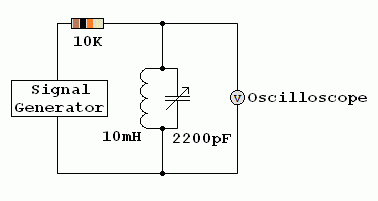c

## Q or Quality Factor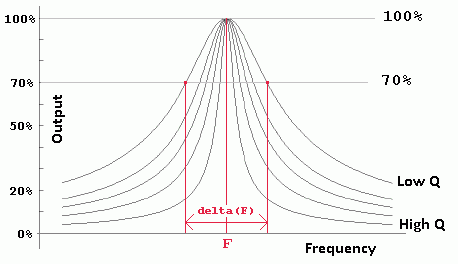• High Q tuned circuits respond to a narrow range of frequencies giving a sharp narrow peak in the graph above.
• Low Q tuned circuits respond to a wider range of frequencies giving a lower and broader peak in the graph above.
• A receiver with a higher Q tuned circuit will be more selective. It will be better at receiving the wanted signal and rejecting signals on adjacent channels.
• Q = F / delta(F).
d

### Below the resonant frequency

• The voltage across the tuned circuit is lower
• The inductor carries most of the current
• The capacitor has less effect
• The current lags behind the applied voltage (is phase shifted by up to 90o)
e

### Above the resonant frequency

• The voltage across the tuned circuit is lower
• The capacitor carries most of the current
• The inductor has less effect
• The current leads the applied voltage (is phase shifted by up to 90o)
f

## At the resonant Frequency

• f is the frequency (Hertz)
• C is the capacitance (Farads)
• L is the inductance (Henrys)

• Xc = XL

• Xc = 1 / ( 2 p f C )

• XL = 2 p f L

• 1 / ( 2 p f C ) = 2 p f L

• f2 = 1 / ( 4 p2 LC )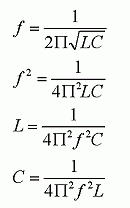• The capacitor is often variable for radio tuning.
• The inductor sometimes has a ferrite or iron core.
• Variable inductors can be used too. These contain a ferrite slug that can be screwed into to the centre of the coil.

Tuned circuits work like this ...

• Energy is stored in the capacitor in the form of electric charge
• Energy is also stored in the inductor in the form of a magnetic field
• When the capacitor is fully charged, there is no current in the inductor
• When the capacitor is fully charged, the potential difference across the circuit is at its maximum
• The potential difference will cause a current to flow in the inductor
• When the current reaches its maximum value, the potential difference across the circuit falls to zero. At this time the magnetic energy stored in the inductor is at its maximum.
• The stored magnetic energy causes the current to continue to flow.
• This current re-charges the capacitor (with opposite polarity).
• When the current falls to zero, the capacitor charge reaches its maximum value.
• This current continues to oscillate back and forth until it dies away due to energy losses.
• An active device such as a field effect transistor can be used boost the energy causing continuous oscillation.

Here is a water analogy.

• The maximum water level difference occurs at the same moment as the minimum water flow.
• The maximum water flow occurs when the water is level and there is no pressure difference between the ends of the bath.
• The water pressure and the water flow are 90 degrees out of phase.

• The same thing happens with the current and voltage in a tuned circuit and a radio antenna.
g

h

## Ferrite Rod Tuned Circuit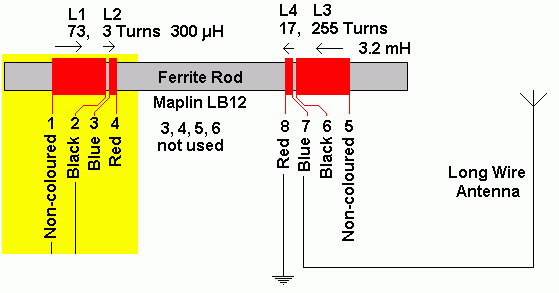This doubles up as the antenna and tuned circuit in low cost radio receivers designed for the long wave (LF) and medium wave (MF) bands.

The addition of the ferrite greatly increases the inductance of the coils. This reduces the resonant frequency.

i

## Ceramic Resonators and Quartz Crystals

These devices also exhibit strong resonant behaviour. They can be used in filter circuits and oscillators replacing the conventional tuned circuit. The advantages are small size, low cost and a high Q factor. However they can't be tuned like an L C circuit.

reviseOmatic V3     Contacts, ©, Cookies, Data Protection and Disclaimers Hosted at linode.com, London